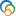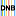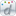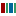The Library of Congress > Linked Data Service > LC Subject Headings (LCSH)

# Newton-Raphson method

• ### Variants

• Method, Newton-Raphson
• Method of tangents
• Newton approximation method
• Newton iterative process
• Newton method
• Newton-Raphson algorithm
• Newton-Raphson formula
• Newton-Raphson process
• Newton's approximation method
• Newton's method
• Raphson method, Newton-
• Second-order Newton-Raphson process

• ### Closely Matching Concepts from Other Schemes

•Newton-Raphson method•Newton-Verfahren•Newton, Méthode de•Newton's methodLabel from public data source Wikidata
• ### Sources

• found: Work cat.: 92-186946: Radzik, T. Newton's method for fractional ... 1992.
• found: Eisenreich. Mathematik:p. 545 (Newton['s] method; Newton-Raphson algorithm, Newton-Raphson formula, Newton-Raphson method, second-order Newton-Raphson process, quadratically convergent Newton-Raphson process, Newton['s] approximation formula)
• found: Math. subj. classif.(49-XX, Calculus of variations and optimal control, Optimization, 49Mxx, Methods of successive approximations; 49M15, Methods of Newton-Raphson, Galerkin, and Ritz types)
• found: Encyc. dict. math.:p. 1125 (Newton-Raphson method; Newton iterative process)
• found: Encyc. math.(Newton method; method of tangents)
• found: CompuMath cit. index.
• found: LC data base, 9/17/92.
• ### Change Notes

• 1992-10-03: new
• 1992-11-16: revised

# Suggest terminology

The LC Linked Data Service welcomes any suggestions you might have about terminology used for a given heading or concept.

Would you like to suggest a change to this heading?

Please provide your name, email, and your suggestion so that we can begin assessing any terminology changes.

Fields denoted with an asterisk (*) are required.